﻿ ﻿ 厦门装饰公司|圣诞狂欢Party等你来嗨！ -- 厦门诚挚装饰设计官网
﻿ ﻿# var time_now_server,time_now_client,time_end,time_server_client,timerID; time_end=new Date("2015/12/25 09:30:50");//结束的时间 time_end=time_end.getTime(); time_now_server=new Date("2015/12/25 09:34:52");//开始的时间 time_now_server=time_now_server.getTime(); time_now_client=new Date(); time_now_client=time_now_client.getTime(); time_server_client=time_now_server-time_now_client; setTimeout("show_time()",1000); function show_time() { var timer = document.getElementById("timer"); if(!timer){ return ; } timer.innerHTML =time_server_client; var time_now,time_distance,str_time; var int_day,int_hour,int_minute,int_second; var time_now=new Date(); time_now=time_now.getTime()+time_server_client; time_distance=time_end-time_now; if(time_distance>0) { int_day=Math.floor(time_distance/86400000) time_distance-=int_day*86400000; int_hour=Math.floor(time_distance/3600000) time_distance-=int_hour*3600000; int_minute=Math.floor(time_distance/60000) time_distance-=int_minute*60000; int_second=Math.floor(time_distance/1000) if(int_hour<10) int_hour="0"+int_hour; if(int_minute<10) int_minute="0"+int_minute; if(int_second<10) int_second="0"+int_second; str_time="剩余时间："+int_day+"天"+int_hour+"小时"+int_minute+"分钟"+int_second+"秒"; timer.innerHTML=str_time; setTimeout("show_time()",1000); } else { timer.innerHTML ="剩余时间：已经结束"; clearTimeout(timerID) } }## 本单详情

【团购内容】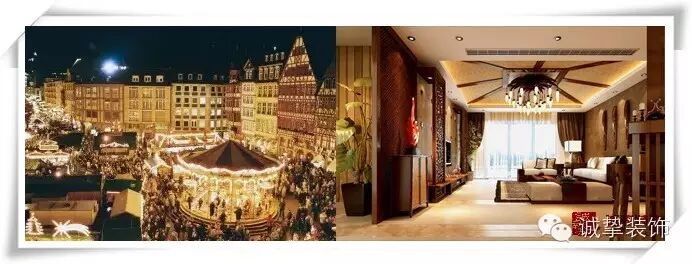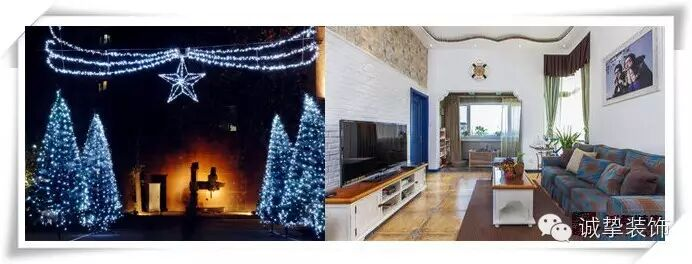地中海风格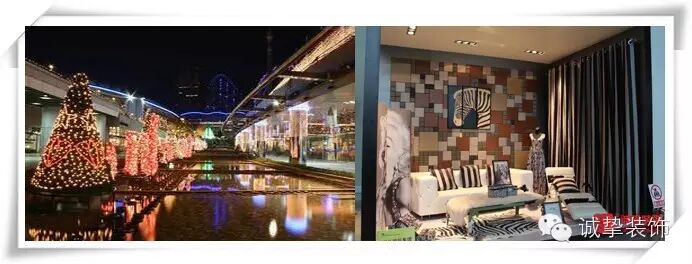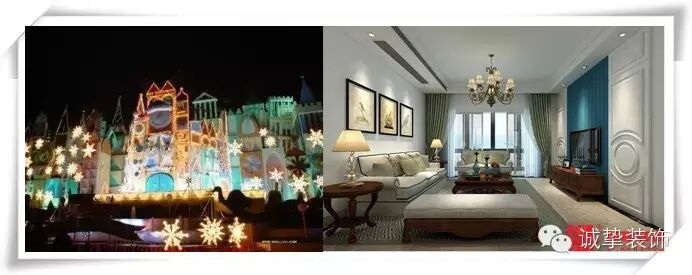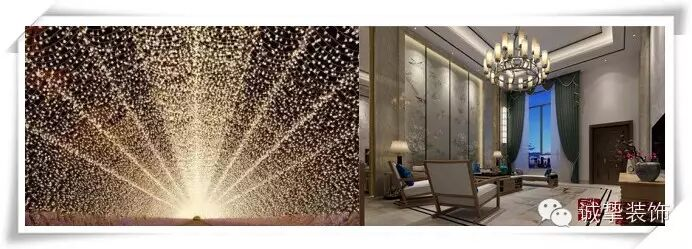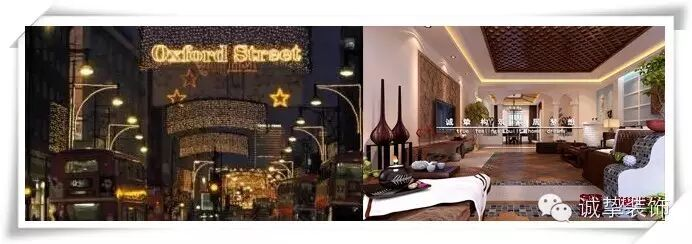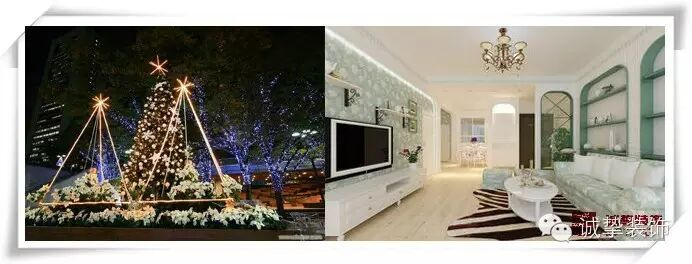田园风格你可以生活的更环保，安全！部分材料展示图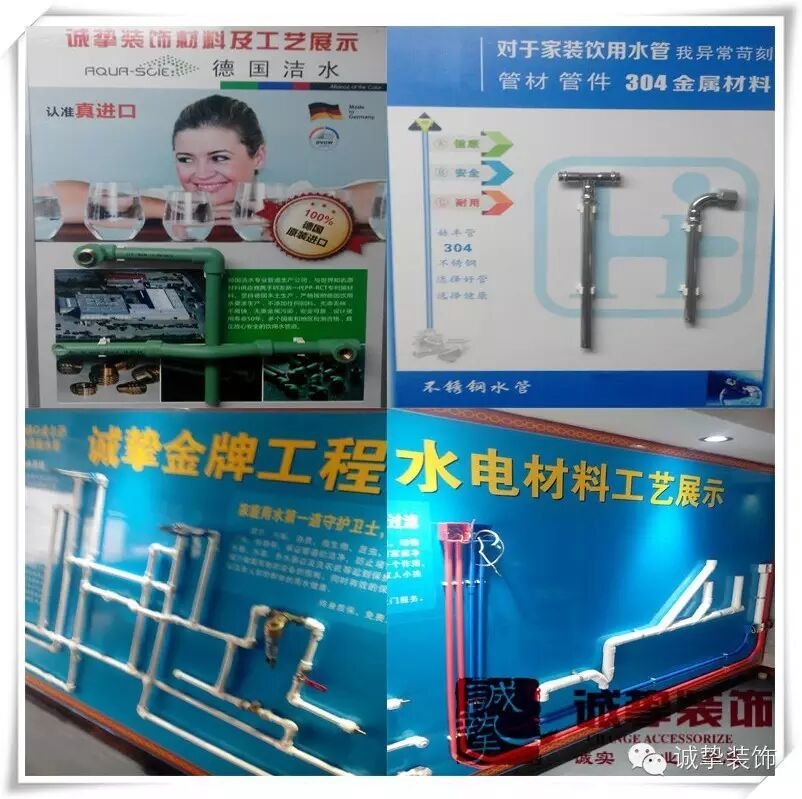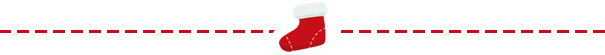Hi~我是厦门装修公司-诚挚装饰您身边的装饰设计服务商

http://www.amoys.com﻿